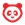cancel
Showing results for
Did you mean:

What-If Grades allow students to calculate their total grade by entering hypothetical grades for assignments. Only students can enter and view What-If scores. Learn how students use What-If Scores.

Notes:

• If Multiple Grading Periods are enabled in your course and users cannot view total grades, or if you have hidden student grade totals in Course Settings, students cannot view their current or total grade in the student Grades page.
• Currently instructors cannot create hypothetical scores for students like What-If scores. However, you can view current and total scores for students by viewing the Student Interactions Report.

Canvas is always working to calculate two grades, the current grade and the total grade, for students. The current grade is calculated by adding up the graded assignments according to their weight in the course grading scheme. This grade is calculated with the Calculate based only on graded assignments checkbox selected in the sidebar.

Note: If you have restricted total grades in Course Settings, your students cannot view their current grade or any percentages in assignment groups.

The total grade is calculated by adding up all the assignments according to their weight in the course grading scheme. If a course is using weighted assignment groups, students can also see how each assignment is weighted in the course.

To view the total grade in the course, students can deselect the Calculate based only on graded assignments checkbox.

Note: If you have restricted total grades in Course Settings, your students cannot view their total grade or any percentages in assignment groups. However, toggling the calculation checkbox still affects individual assignments, such as if an assignment group contains a drop rule.